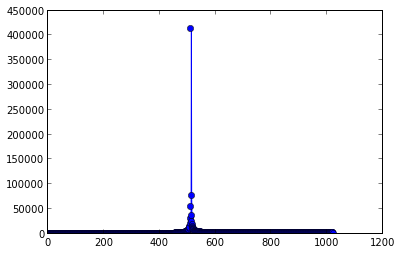$f_{sADC1} = f_{sADC2} = 1 \frac{Msample}{s}$, $t_s = 1.5 \mathrm{cycles}$

Signal generator connected to PA0.

$U_{bias} = \frac{V_{cc}}{2}$

In :
fs = 1e6
nbias = (2**12)/2


## 20 kHz test¶

$f_g = 20 \mathrm{kHz}$, $P_g = 0 \mathrm{dBm}$

In :
fg = 20e3

In :
def load_samples(path):
for line in open(path):
f = line.split()

if f != 'DS':
continue
if f[-1] != 'DE':
continue

x = array(map(float, f[1:-1]))



$N$ - number of samples taken by each ADC.

In :
N = len(x_adc1)

print N

1024


Plot individual samples from both converters.

In :
n_adc1 = arange(1, N*2, 2)

x1, x2, y1, y2 = axis()
axis((0, 50, y1, y2));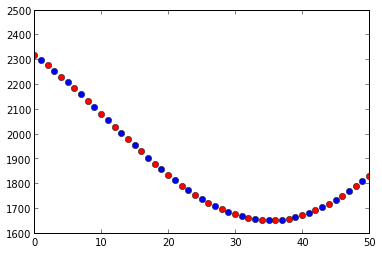Combined output.

In :
x = empty(N*2)

n = arange(0, N*2)

plot(n, x)
grid()
x1, x2, y1, y2 = axis()
axis((0, 100, y1, y2));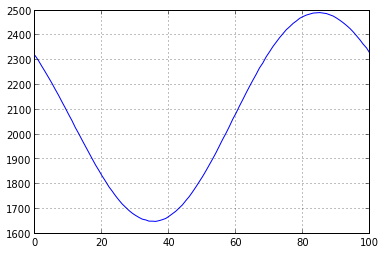Fit an ideal sine wave using only data from ADC1, then only data from ADC2 and compare amplitude, offset, frequency and phase from fitted data.

In :
from scipy.optimize import leastsq

def x_model(c, n):
return c * sin(2. * pi * c * (n + c)) + c

def x_error(c, x, n):
return x_model(c, n) - x

c0 = [ (max(x) - min(x))/2., fg/(2.*fs), -60, mean(x) ]

c = leastsq(x_error, c0, args=(x, n))

xm = x_model(c, n)

x1, x2, y1, y2 = axis()
axis((0, 100, y1, y2))
ylabel("difference from model");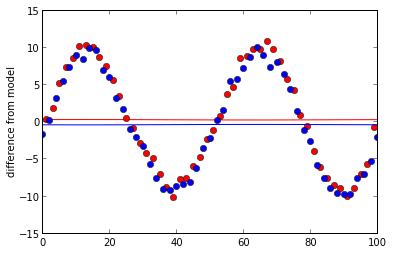Most of the difference we see here is the 2nd harmonic. Either from the signal generator or from non-linearities in the ADC.

In :
dA_adc1, df_adc1, dph_adc1, doff_adc1 = c_adc1 - c


dA_adc1   =  -0.00468228704949

In :
dA_adc2, df_adc2, dph_adc2, doff_adc2 = c_adc2 - c


dA_adc2   =  0.00522541980689


The most important differences here are offset and phase differences. Offset difference comes from calibration differences between converters. Phase difference comes from inaccurate ADC triggering.

Offset between both ADC is less than 1 digit.

Triggering error is on the order of $10^{-3} \frac{1}{f_s}$

### Amplitude accuracy¶

$U_{ef} = \sqrt{P_g \cdot R}$

$U = \sqrt{2} U_{ef}$

$N = \frac{U}{U_{cc}}(2^{12} - 1)$

$N = \frac{\sqrt{2 \cdot 1\mathrm{mW} \cdot 50\mathrm{\Omega}}}{3.3 \mathrm{V}}(2^{12} - 1) = 392$

In :
print "A = ", c

A =  420.77480581


### Frequency accuracy¶

In :
psd = fft.rfft(x)
plot(abs(psd[1:100]), 'o-');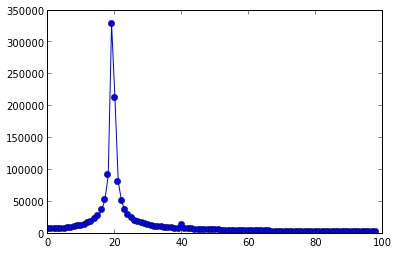## 500 kHz test¶

$f_g = 500 \mathrm{kHz}$, $P_g = 0 \mathrm{dBm}$

In :
x_adc1, x_adc2 = load_samples("test_500khz.log")

x = empty(N*2)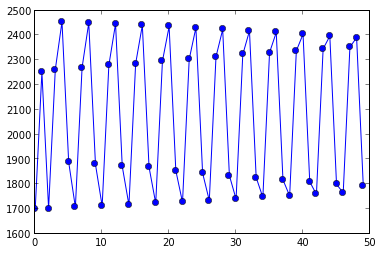psd = fft.rfft(x)